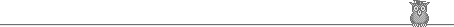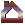# Exercise Set #1

After you have read Lesson 1 try to answer the following exercises.

For each group of sentences fill out the spaces by clicking on the button that corresponds to the right choice from the list at the end of the group. Then peek at the right answers by clicking on the answer button. The correct answer list will appear for as long as you keep clicking on this button.1. ______ μαθητής. (I am a student)
A. B. C. D. E. F.

2. Ο Άγγελος ______ μαθητής. (Angelos is a student)

A. B. C. D. E. F.

3. Εσύ κι εγώ _______ μαθητές (You and I are students)

A. B. C. D. E. F.

 A. είμαι B. είσαι C. είναι D. είμαστε E. είστε F. είναι4. Το βιβλίο ______. (Angelos's book)

A. B. C. D.

5. ______ είναι βλάκας. (Angelos is stupid)

A. B. C. D.

6. Κοίτα _______ ! (See Angelos)

A. B. C. D.

7. Έλα εδώ, _______. (Come here, Angelos)

A. B. C. D.

 A. ο Άγγελος B. του Άγγελου C. τον Άγγελο D. Άγγελε8. Ο Άγγελος δεν είναι ______ θεός. (Angelos is not a god)

A. B. C. D.

9. Ο Άγγελος είναι ______ Θεός. (Angelos is (the) God)

A. B. C. D.

10. Κοίτα _______ Άγγελο! (See Angelos)

A. B. C. D.

11. Έλα εδώ, _______ Άγγελε. (Come here, Angelos)

A. B. C. D.

12. Η Ελλάδα είναι _______ κράτος. (Greece is a state/country)

A. B. C. D.

 A. ο B. το C. τον D. (no article)In general, if the noun is not a proper name or a noun/adjective that in English is translated as "a XXXXXX", then it most probably doesn't take an article. On the other hand, proper names and nouns/adjectives that are translated as "the XXXXX" most of the times do take an article.Main Page